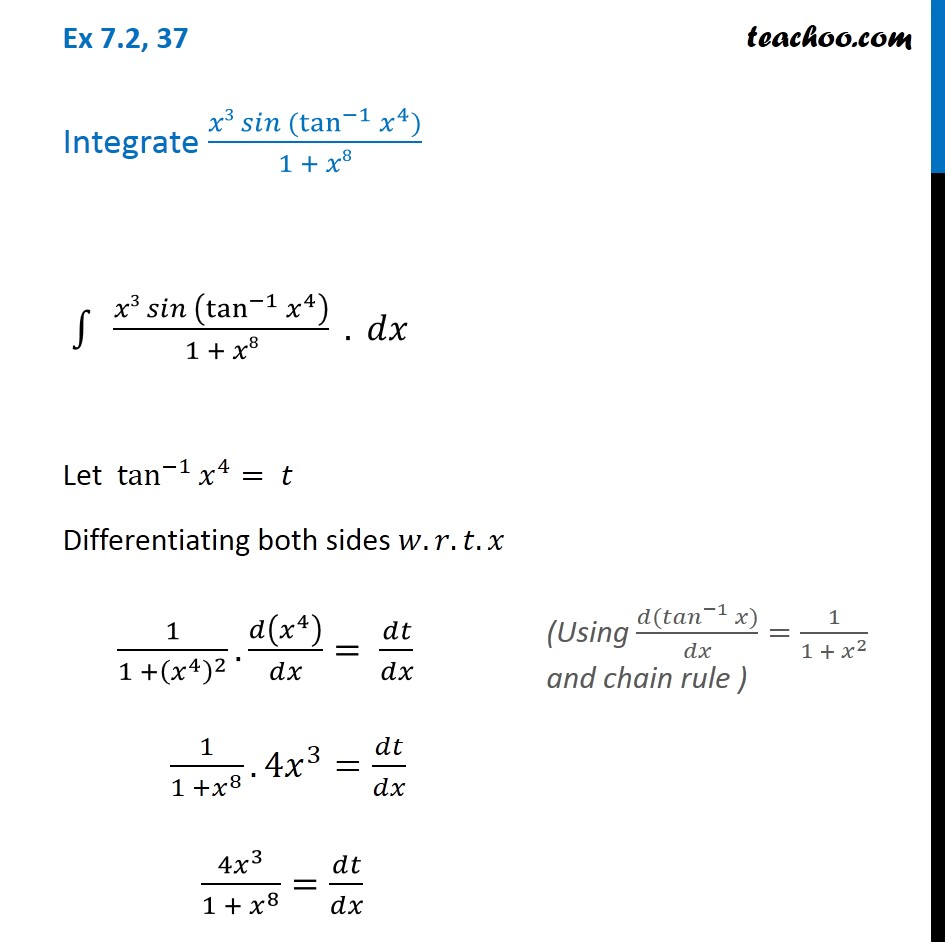Ex 7.2

Chapter 7 Class 12 Integrals (Term 2)
Serial order wise### Transcript

Ex 7.2, 37 Integrate (𝑥3 𝑠𝑖𝑛 (tan^(−1)⁡〖𝑥^4 〗))/(1 + 𝑥8) ∫1▒〖" " (𝑥3 𝑠𝑖𝑛 (tan^(−1)⁡〖𝑥^4 〗 ))/(1 + 𝑥8)〗 . 𝑑𝑥 Let tan^(−1)⁡〖𝑥^4 〗= 𝑡 Differentiating both sides 𝑤.𝑟.𝑡.𝑥 1/(1 +(𝑥^4 )^2 ). 𝑑(𝑥^4 )/𝑑𝑥= 𝑑𝑡/𝑑𝑥 1/(1 +𝑥^8 ). 4𝑥^3=𝑑𝑡/𝑑𝑥 (4𝑥^3)/(1 + 𝑥^8 )=𝑑𝑡/𝑑𝑥 (Using (𝑑(〖𝑡𝑎𝑛〗^(−1)⁡𝑥))/𝑑𝑥=1/(1 + 𝑥^2 ) and chain rule ) 𝑑𝑥=(1 + 𝑥^8)/(4𝑥^3 ) . 𝑑𝑡 Now, our function becomes ∫1▒〖" " (𝑥3 𝑠𝑖𝑛 (tan^(−1)⁡〖𝑥^4 〗 ))/(1 + 𝑥8)〗 . 𝑑𝑥 Putting 〖𝑡𝑎𝑛〗^(−1)⁡〖𝑥^4 〗=𝑡 & 𝑑𝑥=(1 + 𝑥^8)/(4𝑥^3 ) . 𝑑𝑡 = ∫1▒〖" " (𝑥^3 𝑠𝑖𝑛 (𝑡))/(1 + 𝑥^8 )〗. (1 + 𝑥^8)/(4𝑥^3 ) 𝑑𝑡" " = ∫1▒〖" " sin⁡𝑡/4〗 𝑑𝑡" " = 1/4 ∫1▒sin⁡𝑡 . 𝑑𝑡" " = (−1)/4 cos⁡𝑡+ 𝐶 = (−𝟏)/𝟒 〖𝒄𝒐𝒔 〗⁡(〖𝐭𝐚𝐧〗^(−𝟏)⁡〖𝒙^𝟒 〗 )+𝑪 (Using 𝑡=〖𝑡𝑎𝑛〗^(−1)⁡〖𝑥^4 〗)